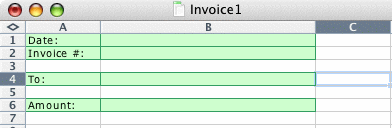#### Mac XL

This site will look better if you upgrade to a browser that supports web standards.

# Using sequential numbers

One common question on the newsgroups is "how do I increment a number in my sheet each time I use it", whether it's an invoice, an order form, or some other numbered form. There are a number of factors to take into account . For instance, whether the current number is saved locally or on a server, or whether more than one person will access a number at a time, or only a single user.

Two ways explored here. It is assumed that the sequential numbers should be stored locally, and that only one number at a time is accessed. The first is to use the registry (and yes, Macs use the equivalent of a registry - the values are stored in file(s) in the Preferences folder). The second uses a text file to store the relevant data. For simplicity I'll assume that an invoice is generated from a template with the following layout:.## Using the registry to hold sequential numbers

An advantage of the registry is that the numbers are unlikely to be inadvertently modified or deleted. A significant disadvantage is that the registry is not designed as a database, which can retain a history, but rather is more suited to storage of a single record. Information is stored in the registry using the SaveSetting method, and retrieved using the GetSetting method. This macro, put in a template's ThisWorkbook code module, will produce an incremented sequential number each time the template is used to generate a document:

```    Private Sub Workbook_Open()
Const sAPPLICATION As String = "Excel"
Const sSECTION As String = "Invoice"
Const sKEY As String = "Invoice_key"
Const nDEFAULT As Long = 1&
Dim nNumber As Long

With ThisWorkbook.Sheets("Invoice")
With .Range("B1")
If IsEmpty(.Value) Then
.Value = Date
.NumberFormat = "dd mmm yyyy"
End If
End With
With .Range("B2")
If IsEmpty(.Value) Then
nNumber = GetSetting(sAPPLICATION, sSECTION, sKEY, nDEFAULT)
.NumberFormat = "@"
.Value = Format(nNumber, "0000")
SaveSetting sAPPLICATION, sSECTION, sKEY, nNumber + 1&
End If
End With
End With
End Sub
```

## Using a text file to hold sequential numbers

This method is more useful in some situations. The biggest advantage is that the sequential number is no longer tied to a particular machine - it can be stored on a common server, or even a thumb drive. Disadvantages include difficulty in keeping the file from being modified simultaneously by two users, or of the file being more easily deleted or modified. This function will return the next sequential number:

```Public Function NextSeqNumber(Optional sFileName As String, Optional nSeqNumber As Long = -1) As Long
Const sDEFAULT_PATH As String = "<your path here>"
Const sDEFAULT_FNAME As String = "defaultseq.txt"
Dim nFileNumber As Long

nFileNumber = FreeFile
If sFileName = "" Then sFileName = sDEFAULT_FNAME
If InStr(sFileName, Application.PathSeparator) = 0 Then _
sFileName = sDEFAULT_PATH & Application.PathSeparator & sFileName
If nSeqNumber = -1& Then
If Dir(sFileName) <> "" Then
Open sFileName For Input As nFileNumber
Input #nFileNumber, nSeqNumber
nSeqNumber = nSeqNumber + 1&
Close nFileNumber
Else
nSeqNumber = 1&
End If
End If
On Error GoTo PathError
Open sFileName For Output As nFileNumber
On Error GoTo 0
Print #nFileNumber, nSeqNumber
Close nFileNumber
NextSeqNumber = nSeqNumber
Exit Function
PathError:
NextSeqNumber = -1&
End Function
```

If you provide a full path in sFileName, that's where the file will be stored. If not, the file will be stored in whatever default directory you specify. You can set the sequential number by providing a value for nSeqNumber.

Thus, if I'm only using one sequence I can use

```    Public Sub Workbook_Open()
ThisWorkbook.Sheets(1).Range("B2").Value = NextSeqNumber
End Sub```

to return the next sequence number. If I'm using multiple sequences, I include the filename (with path, if the text file is not in the default path).

```    Public Sub NewClientInvoice()
ThisWorkbook.Sheets(1).Range("B2").Value = NextSeqNumber("Client1.txt")
End Sub```

And if I want to start a new sequence, beginning at, say, 1001, include that number in the function call. If the client name were in cell B4:

```    Public Sub SetUpNewClient()
With ThisWorkbook.Sheets(1)
.Range("B2").Value = NextSeqNumber(.Range("B4").Value & ".txt", 1001)
End With
End Sub```